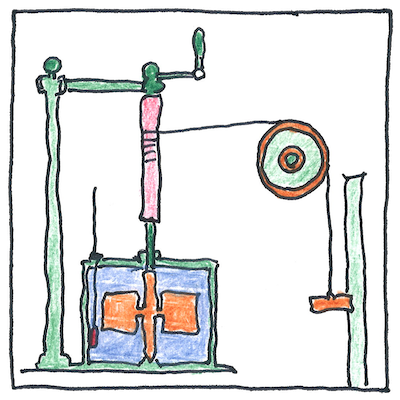# 1842

## The book of science

Tom Sharp

 Julius von Mayer, James Prescott Joule thermodynamics

## Conservation of energy

• In an isolated
• system,
• total energy
• is conserved,
• although it may be
• converted
• from chemical energy
• to kinetic,
• potential, heat, light,
• and sound.

## Independently

• Although not the first to discover
• the relation between heat and mechanical work,
• Julius von Mayer was the first to publish
• a clear quantitative relation between them,
• having discovered that in hotter weather
• his patients’ blood was a deeper red.
• *
• Meanwhile, James Prescott Joule
• built an apparatus that mechanically
• transferred gravitational potential energy
• of a weight into heat and kinetic energy
• of water churned by the weight.

## Not exactly

• Einstein showed the equivalence of energy
• and mass, and we now know that mass may be
• converted to energy; therefore, we speak
• of the conservation of mass-energy, except
• for systems without time translation symmetry,
• in which case even perpetual motion happens.

## Perpetual entropy

• Potential energy
• Perpetual entropy
• Hypothesized ocean
• Actualized emotion
• Imperpetual motion
• Intellectual commotion

Galileo’s analysis of “interrupted pendulum” in 1638, Christian Huygens’s laws of collision in 1669, Gottfried Wilhelm Leibniz’s conservation of mass times velocity (in systems where the masses don’t interact) between 1676 and 1689, Isaac Newton’s assumption of the exact conservation of mechanical momentum in 1687, Johann Bernoulli’s law of conservation of vis viva in 1715, Daniel Bernoulli’s principle of virtual work in 1738, Émilie du Châtelet (1706 – 1749) hypothesis of the conservation of total energy, as distinct from momentum, in 1740, the Lagrange-D’Alembert’s principle of classical mechanics (a general case of Newton’s second law, F=ma) in 1742, all led to understanding energy and the laws of thermodynamics.